xCrystallographic point groupEncyclopedia
In crystallography
Crystallography
Crystallography is the experimental science of the arrangement of atoms in solids. The word "crystallography" derives from the Greek words crystallon = cold drop / frozen drop, with its meaning extending to all solids with some degree of transparency, and grapho = write.Before the development of...

, a crystallographic point group is a set of symmetry
Symmetry
Symmetry generally conveys two primary meanings. The first is an imprecise sense of harmonious or aesthetically pleasing proportionality and balance; such that it reflects beauty or perfection...

operations, like rotations or reflections, that leave a central point fixed while moving other directions and faces of the crystal
Crystal
A crystal or crystalline solid is a solid material whose constituent atoms, molecules, or ions are arranged in an orderly repeating pattern extending in all three spatial dimensions. The scientific study of crystals and crystal formation is known as crystallography...

to the positions of features of the same kind. For a true crystal (as opposed to a quasicrystal
Quasicrystal
A quasiperiodic crystal, or, in short, quasicrystal, is a structure that is ordered but not periodic. A quasicrystalline pattern can continuously fill all available space, but it lacks translational symmetry...

), the group must also be consistent with maintenance of the three-dimensional translational symmetry
Translational symmetry
In geometry, a translation "slides" an object by a a: Ta = p + a.In physics and mathematics, continuous translational symmetry is the invariance of a system of equations under any translation...

that defines crystallinity. The macroscopic properties of a crystal would look exactly the same before and after any of the operations in its point group. In the classification of crystals, each point group is also known as a crystal class.

There are infinitely many three-dimensional point groups; However, the crystallographic restriction
Crystallographic restriction theorem
The crystallographic restriction theorem in its basic form was based on the observation that the rotational symmetries of a crystal are usually limited to 2-fold, 3-fold, 4-fold, and 6-fold...

of the infinite families of general point group
Point group
In geometry, a point group is a group of geometric symmetries that keep at least one point fixed. Point groups can exist in a Euclidean space with any dimension, and every point group in dimension d is a subgroup of the orthogonal group O...

s results in there being only 32 crystallographic point groups. These 32 point groups are one-and-the same as the 32 types of morphological (external) crystalline symmetries derived in 1830 by Johann Friedrich Christian Hessel
Johann F. C. Hessel
Johann Friedrich Christian Hessel was a German physician and professor of mineralogy at the University of Marburg....

from a consideration of observed crystal forms.

The point group of a crystal, among other things, determines directional variation of the physical properties that arise from its structure, including optical properties
Crystal optics
Crystal optics is the branch of optics that describes the behaviour of light in anisotropic media, that is, media in which light behaves differently depending on which direction the light is propagating. The index of refraction depends on both composition and crystal structure and can be...

such as whether it is birefringent
Birefringence
Birefringence, or double refraction, is the decomposition of a ray of light into two rays when it passes through certain anisotropic materials, such as crystals of calcite or boron nitride. The effect was first described by the Danish scientist Rasmus Bartholin in 1669, who saw it in calcite...

, or whether it shows the Pockels effect
Pockels effect
The Pockels effect , or Pockels electro-optic effect, produces birefringence in an optical medium induced by a constant or varying electric field. It is distinguished from the Kerr effect by the fact that the birefringence is proportional to the electric field, whereas in the Kerr effect it is...

.

## Notation

The point groups are denoted by their component symmetries. There are a few standard notations used by crystallographers, mineralogists, and physicists.

For the correspondence of the two systems below, see crystal system
Crystal system
In crystallography, the terms crystal system, crystal family, and lattice system each refer to one of several classes of space groups, lattices, point groups, or crystals...

.

### Schönflies notation

In Schönflies
Arthur Moritz Schönflies
Arthur Moritz Schoenflies , sometimes written as Schönflies, was a German mathematician, known for his contributions to the application of group theory to crystallography, and for work in topology....

notation, point groups are denoted by a letter symbol with a subscript. The symbols used in crystallography mean the following:
• Cn (for cyclic
Cyclic group
In group theory, a cyclic group is a group that can be generated by a single element, in the sense that the group has an element g such that, when written multiplicatively, every element of the group is a power of g .-Definition:A group G is called cyclic if there exists an element g...

) indicates that the group has an n-fold rotation axis. Cnh is Cn with the addition of a mirror (reflection) plane perpendicular to the axis of rotation. Cnv is Cn with the addition of a mirror plane parallel to the axis of rotation.
• S2n (for Spiegel, German for mirror
Mirror
A mirror is an object that reflects light or sound in a way that preserves much of its original quality prior to its contact with the mirror. Some mirrors also filter out some wavelengths, while preserving other wavelengths in the reflection...

) denotes a group that contains only a 2n-fold rotation-reflection axis.
• Dn (for dihedral
Dihedral group
In mathematics, a dihedral group is the group of symmetries of a regular polygon, including both rotations and reflections. Dihedral groups are among the simplest examples of finite groups, and they play an important role in group theory, geometry, and chemistry.See also: Dihedral symmetry in three...

, or two-sided) indicates that the group has an n-fold rotation axis plus a twofold axis perpendicular to that axis. Dnh has, in addition, a mirror plane perpendicular to the n-fold axis. Dnd has, in addition to the elements of Dn, mirror planes parallel to the n-fold axis.
• The letter T (for tetrahedron
Tetrahedron
In geometry, a tetrahedron is a polyhedron composed of four triangular faces, three of which meet at each vertex. A regular tetrahedron is one in which the four triangles are regular, or "equilateral", and is one of the Platonic solids...

) indicates that the group has the symmetry of a tetrahedron. Td includes improper rotation operations, T excludes improper rotation operations, and Th is T with the addition of an inversion.
• The letter O (for octahedron
Octahedron
In geometry, an octahedron is a polyhedron with eight faces. A regular octahedron is a Platonic solid composed of eight equilateral triangles, four of which meet at each vertex....

) indicates that the group has the symmetry of an octahedron (or cube
Cube
In geometry, a cube is a three-dimensional solid object bounded by six square faces, facets or sides, with three meeting at each vertex. The cube can also be called a regular hexahedron and is one of the five Platonic solids. It is a special kind of square prism, of rectangular parallelepiped and...

), with (Oh) or without (O) improper operations (those that change handedness).

Due to the crystallographic restriction theorem
Crystallographic restriction theorem
The crystallographic restriction theorem in its basic form was based on the observation that the rotational symmetries of a crystal are usually limited to 2-fold, 3-fold, 4-fold, and 6-fold...

, n = 1, 2, 3, 4, or 6 in 2- or 3-dimensional space.
n 1 2 3 4 6
Cn C1 C2 C3 C4 C6
Cnv C1v=C1h C2v C3v C4v C6v
Cnh C1h C2h C3h C4h C6h
Dn D1=C2 D2 D3 D4 D6
Dnh D1h=C2v D2h D3h D4h D6h
Dnd D1d=C2h D2d D3d D4d D6d
S2n S2 S4 S6 S8 S12

D4d and D6d are actually forbidden because they contain improper rotation
Improper rotation
In 3D geometry, an improper rotation, also called rotoreflection or rotary reflection is, depending on context, a linear transformation or affine transformation which is the combination of a rotation about an axis and a reflection in a plane perpendicular to the axis.Equivalently it is the...

s with n=8 and 12 respectively. The 27 point groups in the table plus T, Td, Th, O and Oh constitute 32 crystallographic point groups

### Hermann–Mauguin notation

An abbreviated form of the Hermann–Mauguin notation commonly used for space group
Space group
In mathematics and geometry, a space group is a symmetry group, usually for three dimensions, that divides space into discrete repeatable domains.In three dimensions, there are 219 unique types, or counted as 230 if chiral copies are considered distinct...

s also serves to describe crystallographic point groups. Group names are
 1 2 2⁄m 222 m mm2 mmm 3 32 3m m 4 4⁄m 422 4mm 2m 4⁄mmm 6 6⁄m 622 6mm 2m 6⁄mmm 23 m 432 3m mm

### The correspondence between different notations

 Crystal familyCrystal systemIn crystallography, the terms crystal system, crystal family, and lattice system each refer to one of several classes of space groups, lattices, point groups, or crystals...  Crystal systemCrystal systemIn crystallography, the terms crystal system, crystal family, and lattice system each refer to one of several classes of space groups, lattices, point groups, or crystals...  Hermann-Mauguin(full symbol) Hermann-Mauguin(short symbol) Shubnikov Schoenflies Orbifold CoxeterCoxeter notationIn geometry, Coxeter notation is a system of classifying symmetry groups, describing the angles between with fundamental reflections of a Coxeter group. It uses a bracketed notation, with modifiers to indicate certain subgroups. The notation is named after H. S. M... Order TriclinicTriclinic crystal systemthumb|180px|Triclinic In crystallography, the triclinic crystal system is one of the 7 crystal systems. A crystal system is described by three basis vectors. In the triclinic system, the crystal is described by vectors of unequal length, as in the orthorhombic system... 1 1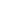C1 11 [ ]+ 1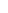Ci = S2 x [1+,2+] 2 MonoclinicMonoclinic crystal systemIn crystallography, the monoclinic crystal system is one of the 7 lattice point groups. A crystal system is described by three vectors. In the monoclinic system, the crystal is described by vectors of unequal length, as in the orthorhombic system. They form a rectangular prism with a... 2 2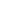C2 22 + 2 m mCs = C1h * [ ] 2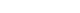2/m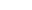C2h 2* [2,2+] 4 OrthorhombicOrthorhombic crystal systemIn crystallography, the orthorhombic crystal system is one of the seven lattice point groups. Orthorhombic lattices result from stretching a cubic lattice along two of its orthogonal pairs by two different factors, resulting in a rectangular prism with a rectangular base and height , such that a,... 222 222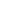D2 = V 222 [2,2]+ 4 mm2 mm2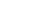C2v *22  4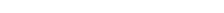mmm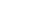D2h *222 [2,2] 8 TetragonalTetragonal crystal systemIn crystallography, the tetragonal crystal system is one of the 7 lattice point groups. Tetragonal crystal lattices result from stretching a cubic lattice along one of its lattice vectors, so that the cube becomes a rectangular prism with a square base and height .There are two tetragonal Bravais... 4 4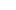C4 44 + 4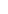S4 2x [2+,4+] 4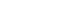4/m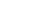C4h 4* [2,4+] 8 422 422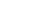D4 422 [4,2]+ 8 4mm 4mm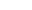C4v *44  8 2m 2m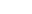D2d 2*2 [2+,4] 8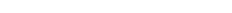4/mmm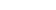D4h *422 [4,2] 16 HexagonalHexagonal crystal systemIn crystallography, the hexagonal crystal system is one of the 7 crystal systems, the hexagonal lattice system is one of the 7 lattice systems, and the hexagonal crystal family is one of the 6 crystal families... Trigonal 3 3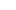C3 33 + 3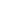S6 = C3i 3x [2+,6+] 6 32 32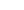D3 322 [3,2]+ 6 3m 3m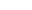C3v *33  6m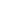D3d 2*3 [2+,6] 12 HexagonalHexagonal crystal systemIn crystallography, the hexagonal crystal system is one of the 7 crystal systems, the hexagonal lattice system is one of the 7 lattice systems, and the hexagonal crystal family is one of the 6 crystal families... 6 6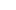C6 66 + 6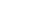C3h 3* [2,3+] 6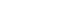6/m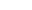C6h 6* [2,6+] 12 622 622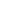D6 622 [6,2]+ 12 6mm 6mm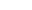C6v *66  12 m2 m2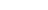D3h *322 [3,2] 12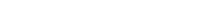6/mmm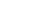D6h *622 [6,2] 24 CubicCubic crystal systemIn crystallography, the cubic crystal system is a crystal system where the unit cell is in the shape of a cube. This is one of the most common and simplest shapes found in crystals and minerals.... 23 23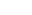T 332 [3,3]+ 12m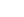Th 3*2 [3+,4] 24 432 432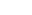O 432 [4,3]+ 24 3m 3m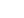Td *332 [3,3] 24mm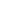Oh *432 [4,3] 48

• Molecular symmetry
Molecular symmetry
Molecular symmetry in chemistry describes the symmetry present in molecules and the classification of molecules according to their symmetry. Molecular symmetry is a fundamental concept in chemistry, as it can predict or explain many of a molecule's chemical properties, such as its dipole moment...

• Point group
Point group
In geometry, a point group is a group of geometric symmetries that keep at least one point fixed. Point groups can exist in a Euclidean space with any dimension, and every point group in dimension d is a subgroup of the orthogonal group O...

• Space group
Space group
In mathematics and geometry, a space group is a symmetry group, usually for three dimensions, that divides space into discrete repeatable domains.In three dimensions, there are 219 unique types, or counted as 230 if chiral copies are considered distinct...

• Point groups in three dimensions
Point groups in three dimensions
In geometry, a point group in three dimensions is an isometry group in three dimensions that leaves the origin fixed, or correspondingly, an isometry group of a sphere. It is a subgroup of the orthogonal group O, the group of all isometries that leave the origin fixed, or correspondingly, the group...

• Crystal system
Crystal system
In crystallography, the terms crystal system, crystal family, and lattice system each refer to one of several classes of space groups, lattices, point groups, or crystals...